# Water solids concentration

Jump to: navigation, search

## Water solids concentration

The concentration of solids in formation water (brines) are reported in several different ways .

## Math and Physics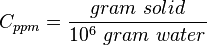$C_{ppm} = \frac{gram\ solid}{10^6\ gram\ water}$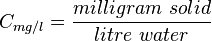$C_{mg/l} = \frac{milligram\ solid}{litre\ water}$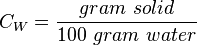$C_W = \frac{gram\ solid}{100\ gram\ water}$

## Conversions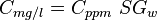$C_{mg/l} = C_{ppm}\ SG_w$

Often, parts per million and milligrams per liter are used interchangeably. This is correct only if the density of the brine at standard conditions can be assumed to be 1 g/cc ( 1000 kg/m3, SG_w=1, 62.428 lbm/ft3) .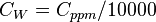$C_W = C_{ppm}/10000$

## Nomenclature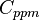$C_{ppm}$ = water salinity or solids concentration, ppm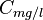$C_{mg/l}$ = water salinity or solids concentration, mg/l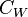$C_W$ = water salinity or weight percent solids, %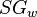$SG_w$ = water specific gravity, dimensionless

## See also

Water bubble point pressure
Water compressibility
Water density
Water formation volume factor
Water salinity from density equation
Water solids concentration
Water viscosity
Gas/Water Interfacial Tension